Courses

# Kinetic Theory Of Gases NAT Level – 1

## 10 Questions MCQ Test Topic wise Tests for IIT JAM Physics | Kinetic Theory Of Gases NAT Level – 1

Description
This mock test of Kinetic Theory Of Gases NAT Level – 1 for Physics helps you for every Physics entrance exam. This contains 10 Multiple Choice Questions for Physics Kinetic Theory Of Gases NAT Level – 1 (mcq) to study with solutions a complete question bank. The solved questions answers in this Kinetic Theory Of Gases NAT Level – 1 quiz give you a good mix of easy questions and tough questions. Physics students definitely take this Kinetic Theory Of Gases NAT Level – 1 exercise for a better result in the exam. You can find other Kinetic Theory Of Gases NAT Level – 1 extra questions, long questions & short questions for Physics on EduRev as well by searching above.
*Answer can only contain numeric values
QUESTION: 1

### The capacity of a vessel in 3 litres. If contains 6gm oxygen, 8gm nitrogen and 5gm CO2 mixture at 27°C. If R = 7.31 J/mole-K, the pressure the vessel in units of 103 N/m2 will be

Solution:

p = p1 + p2 + p3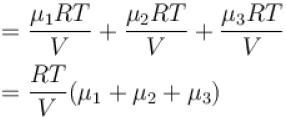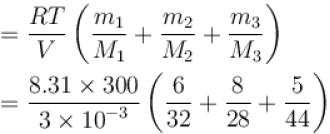= 500 × 103 N/m2

*Answer can only contain numeric values
QUESTION: 2

### 22gm of CO2 at 27°C is mixed with 16 gm of O2 at 37°C. The temperature of the mixture (in °C) is?

Solution:

Let t be the temperature of mixture
Heat gained by CO2 = Heat lost by O2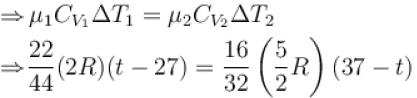⇒ t = 32°C

*Answer can only contain numeric values
QUESTION: 3

### N molecule each of mass m of gas A and 2N molecules each of mass 2m of gas B are contained in the some vessel at temperature T. The mean square at temperature T. The mean square of the velocity of molecules of gas B is v2 and the mean square of x component of the velocity of molecules of gas A is w2 . The ratio w2/v2 upto 2 decimal plane is.

Solution:

Mean square velocity of molecule = 3kT/m
For gas A, x component of mean square velocity of molecule = w2    ...(1)
∴ Mean square velocity =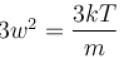...(2)
For B gas, mean square velocity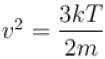...(3)
From (1) and (2),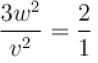So,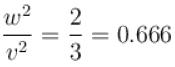= 0.67

*Answer can only contain numeric values
QUESTION: 4

The gases occupy two containers A and B. Gas in A, of volume 0.1 m3 exerts a pressure of 1.40 MPa and that in B of volume 0.15 m2 exerts a pressure 0.7 MPa. The two containers are united by a tube of negligible volume and gases are allowed to intermingle. Then if the temperature remains constant the final pressure in the container will be (in MPa)

Solution:

µA + µB = µ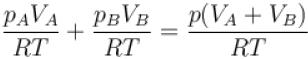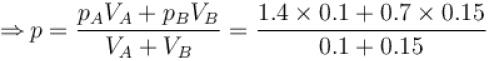p = 0.98MPa

*Answer can only contain numeric values
QUESTION: 5

A gas mixture consists of 2 mole of oxygen and 4 mole of argon at temperature T. Neglecting all vibrational modes, the total internal energy of the system in the units of RT is given as?

Solution:

Total internal energy of system
= Uoxygen + Uargon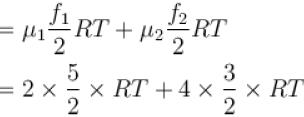= 11RT (f1 = 5 for oxygen and f2 = 3 for argon.)

*Answer can only contain numeric values
QUESTION: 6

At standard temperature and pressure, the density of a gas is 1.3 kg/m3 and the speed of the sound and the speed of the sound in gas is 330 m/s. Then the degree of freedom of the gas will be?

Solution:

Velocity of sound  vs = 330 m/s
Density of gas ρ = 1.5kg/m3
Atmosphere Pressure p = 1.01 × 105 N/m2
Substituting these values in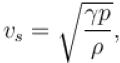we get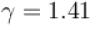Now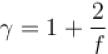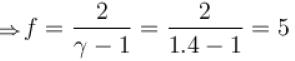*Answer can only contain numeric values
QUESTION: 7

The mean free path of nitrogen molecules at a pressure of 1.0 atm and temperature 0°C is 0.8 × 10-7 m. If the number density of molecules is 2.7 × 1025 /m3 , then the molecular diameter (in Å) is.

Solution: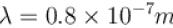n = 2.7 × 1025 /m3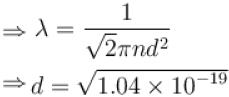d = 3.2 × 10-10 m
⇒ d = 3.2Å

*Answer can only contain numeric values
QUESTION: 8

The root mean square speed of hydrogen molecules of an ideal hydrogen gas kept in a gas chamber at 0°C is 3180 m/s. The pressure of hydrogen gas (in atm) is?
(density of hydrogen gas is 8.99 × 10-2 kg/m3 , 1 atm = 1.0 × 105 N/m2 )

Solution:

As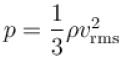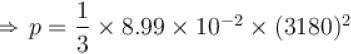p = 3.03 × 105 N/m2
p = 3 atm

*Answer can only contain numeric values
QUESTION: 9

A cylinder of capacity 20 litres is filled with H2 gas. The total average kinetic energy of translatory motion of its molecules is 1.5 × 105 J. The pressure of hydrogen in the cylinder is (in 106 N/m2).

Solution:

Kinetic Energy E = 1.5 × 105J
Volume V = 20 litres = 20 × 10-3 m3
Pressure =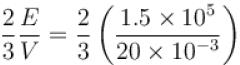p = 5 × 106 N/m2

*Answer can only contain numeric values
QUESTION: 10

If the mean free path of atoms is doubled, then the pressure of gas will change by what multiplicative factor?

Solution:

As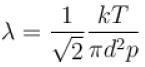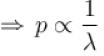i.e. by increasing ρ two times, pressure will become half.